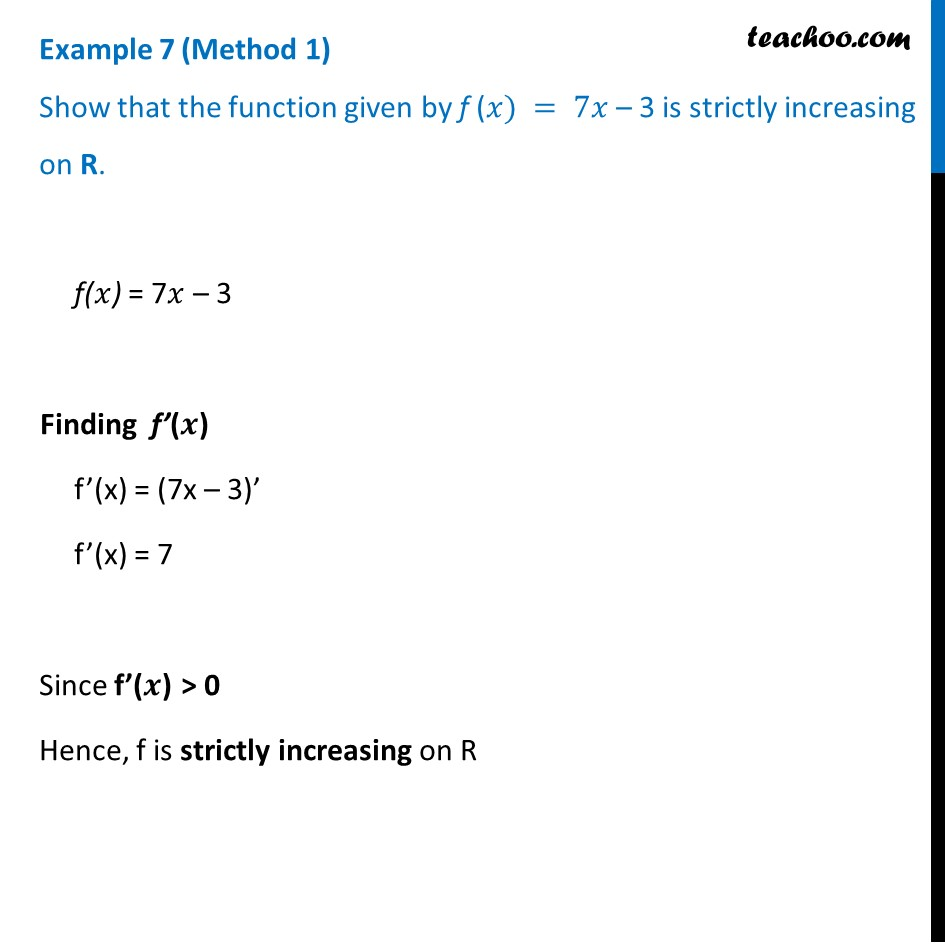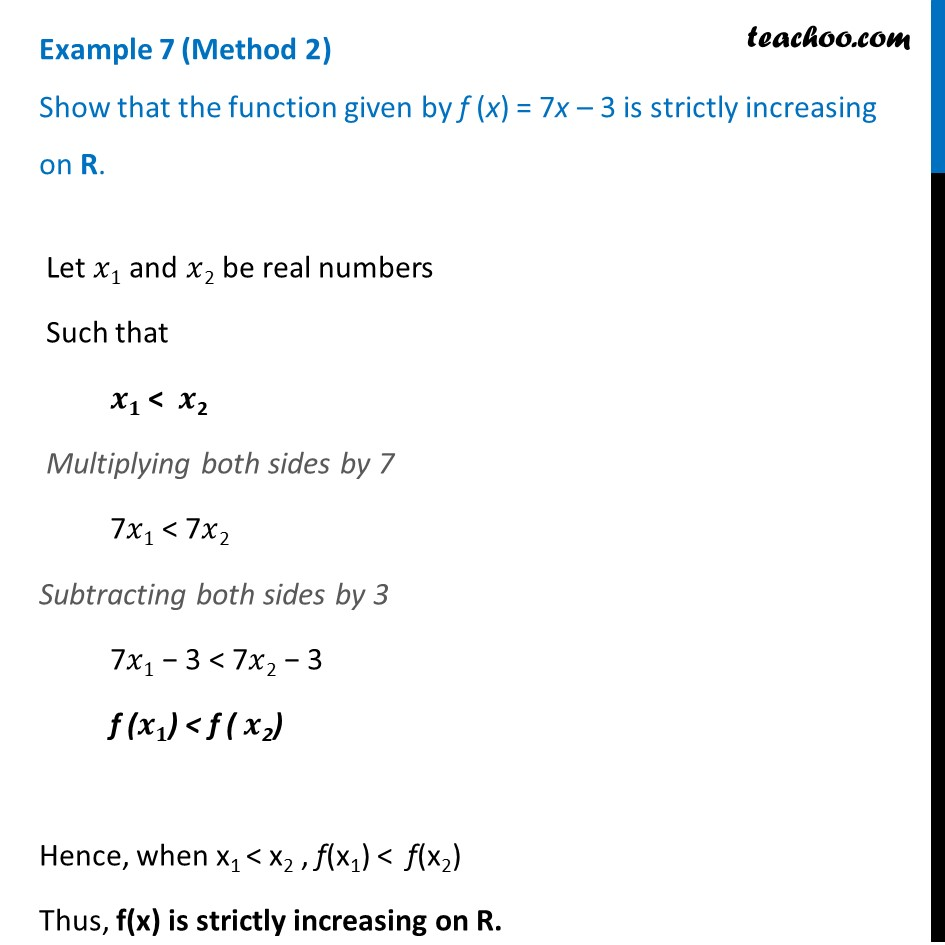To show increasing/decreasing in whole domain

Chapter 6 Class 12 Application of Derivatives
Concept wiseLearn in your speed, with individual attention - Teachoo Maths 1-on-1 Class

### Transcript

Example 7 (Method 1) Show that the function given by f (𝑥) = 7𝑥 – 3 is strictly increasing on R.f(𝑥) = 7𝑥 – 3 Finding f’(𝒙) f’(x) = (7x – 3)’ f’(x) = 7 Since f’(𝒙) > 0 Hence, f is strictly increasing on R Example 7 (Method 2 ) Show that the function given by f (x) = 7x – 3 is strictly increasing on R. Let 𝑥1 and 𝑥2 be real numbers Such that 𝒙𝟏 < 𝒙2 Multiplying both sides by 7 7𝑥1 < 7𝑥2 Subtracting both sides by 3 7𝑥1 − 3 < 7𝑥2 − 3 f (𝒙𝟏) < f ( 𝒙2) Hence, when x1 < x2 , f(x1) < f(x2) Thus, f(x) is strictly increasing on R.